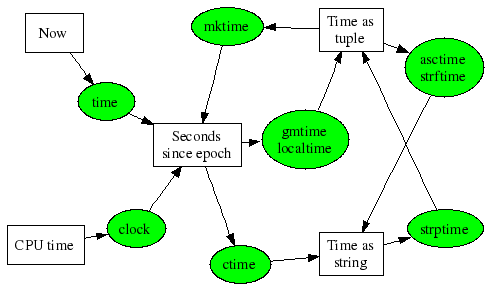# Time Functions## Motivation

Working in software I'm constantly having to look up the functions in C, Python, etc., for converting between different representations of time. So I made up this chart, printed it, and put it on the wall.

Saves me time ...

## Comment

I know "Seconds since epoch" is a bit odd for the destination of "clock", but in another sense it's right. It's the number of seconds this process has used, and that's since the process was started. But it didn't use any before it was started, so although "since epoch" is a bit misleading, it's nonetheless right.

## strXtime codes

 %a/%A Locale's weekday name, abbr/full %b/%B Locale's month name, abbr/full %c Locale's appropriate date and time representation. %d Day of the month as a decimal number [01,31]. %f Microsecond as a decimal number [0,999999], zero-padded on the left %H Hour (24-hour clock) as a decimal number [00,23]. %I Hour (12-hour clock) as a decimal number [01,12]. %j Day of the year as a decimal number [001,366]. %m Month as a decimal number [01,12]. %M Minute as a decimal number [00,59]. %p Locale's equivalent of either AM or PM. %S Second as a decimal number [00,61]. %U Week number of the year (Sunday as the first day of the week) as a decimal number [00,53]. All days in a new year preceding the first Sunday are considered to be in week 0. %w Weekday as a decimal number [0(Sunday),6]. %W Week number of the year (Monday as the first day of the week) as a decimal number [00,53]. All days in a new year preceding the first Monday are considered to be in week 0. %x Locale's appropriate date representation. %X Locale's appropriate time representation. %y Year without century as a decimal number [00,99]. %Y Year with century as a decimal number. %z UTC offset in the form +HHMM or -HHMM (empty string if the the object is naive). %Z Time zone name (empty string if the object is naive). %% A literal '%' character.

# ContentsSuggest a change ( <-- What does this mean?) / Send me email